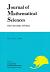## Vol. 19 (2012) No. 04Journal of Mathematical Sciences The University of Tokyo

1. Goto, Satoshi
On the Fusion Algebras of Bimodules Arising from Goodman-de la Harpe-Jones Subfactors
Vol. 19 (2012), No. 4, Page 409-506.

2. Asai, Tomoro
Quasilinear parabolic equation and its applications to fourth order equations with rough initial data
Vol. 19 (2012), No. 4, Page 507–532.

3. KUSUOKA, Shigeo
A remark on Malliavin Calculus : Uniform Estimates and Localization
Vol. 19 (2012), No. 4, Page 533–558.

4. Honda, Naofumi ; Umeta, Kohei
On the sheaf of Laplace hyperfunctions with holomorphic parameters
Vol. 19 (2012), No. 4, Page 559–586.

5. Gomi, Kiyonori
Clifford modules, finite-dimensional approximation and twisted $K$-theory
Vol. 19 (2012), No. 4, Page 587–612.

6. Nakayama, Chikara
Log Néron models over surfaces
Vol. 19 (2012), No. 4, Page 613–659.

7. Noguchi, Junjiro
Another Direct Proof of Oka's Theorem (Oka IX)
Vol. 19 (2012), No. 4, Page 661–675.
Lower weight Gel'fand-Kalinin-Fuks cohomology groups of the formal Hamiltonian vector fields on $\mathbb R^4$